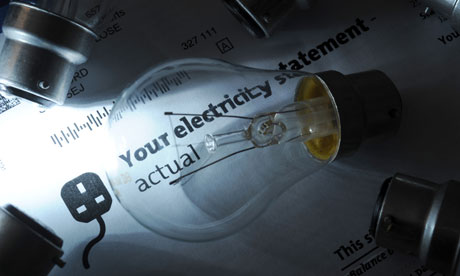# A simple guide to calculating your UMEME YAKA Electricity BillIf those figures on your Yaka meter have been mysterious to you or you probably think UMEME is cheating you, well, it’s time to get your hands dirty. It’s a bit technical. But like all things, it becomes easy if you understand the jargon and follow through.

## The basics: Understanding the units of electricity

The most basic units of measuring electricity are Voltage(V) and Current(I). Voltage is measured in Volts(V)s while current is measured in Amps (A). So what’s voltage and current? We don’t have time to rebound a physics class, so a familiar analogy of plumbing would suffice.

Think of water flowing from a tank at your home. The voltage is equivalent to the water pressure while current is equivalent to the rate at which water flows. Sounds simple now, right? Increase the water pressure(Voltage) and more water (Current) will gush out as you would expect.

So if you are a domestic user, your voltage is 240V for one phase. Industries need more power and are therefore supplied with three phases. Think of phases as being the different channels or pipes through which the water flows. The more pipes, the more water.

Now, Electrical power is measured in watts(W). It’s a result of multiplying Voltage(V) and Current(I). Again, using our analogy, we can have more power if we increase the water pressure or the water flow rate. Energy is the amount of power(Watts) used over a period of time(Hours). As you have guessed energy units are Watt Hours (Wh) and this is what you pay for on your monthly Umeme Yaka electricity bill.

## Before we start crunching your bill

1.  Check the back plate of your appliance for the its electrical power rating in Watts (W). This is a measure of power that your appliance uses. The higher the figure, the more power your appliance uses and the bigger your power bill will be.

2.  Estimate the usage (hours/time) of each appliance in your house. Find out on average how many hours(h) you use it per day. Then estimate the number of days in a month you use the appliance. The more hours and days you use your appliance, the bigger your power bill.

3. A unit of energy is measured in Kilo Watt Hours (kWh). That’s got by multiplying the  power(W) of our appliance to time of use in hours (h) divided by 1,000 — remember kilo means 1,000.

4. Take logs of your meter reading so that you can compare notes with UMEME. We have written a comprehensive guide on how to use your Yaka prepaid meter, so those tips will come in handy.

## UMEME Yaka power rates and billing

Based on the latest UMEME power tariff structure (10th July 2019);

1. Umeme charges the first 15 units that you consume UGX 250 per unit.

2. The proceeding units are charged UGX 755.1 per unit.

3. Then there’s a monthly service fee of UGX 3,360

4. Umeme then charges 18% on VAT.

## How to Calculate

We will give a nice easy example so that everything else below makes sense. Lets use a Flat Iron as an example. If your flat iron uses 1000W (You can check on the back to see the wattage of your appliance)  and you use it for 1 hour, You will get the wattage (1000W) multiply it by the number of hours used eg. 1 hour and divide it by 1000.

If you got the calculation right, then the answer should be 1 which is 1KWH. So if you use your 1000W flat iron for an hour, you would have used 1kWh  ( or 1 unit) of electricity. You can further confirm this by noting the number of units on your Customer Interface Unit (CIU) or the Yaka meter as we commonly know it before using the 1000W flat iron and note the units left after using it for one hour. As you can see it’s not hard at all to do so let’s get a more applicable example below.

## Home appliances and average usage

So let’s say your house has;

1.  6 Philips energy savers each with a power rating of 11W. Let’s say you use them for 6 hours a day.

2.  1 Philips flat iron of a power rating of 1,000W. You iron for half an hour a day

3.  1 LG Microwave of 2,000 W which you use for quarter an hour a day.

4.  1 Samsung UN32EH4000 LED 32 inch Flat screen TV with power of 29 Watt. You watch TV for 4 hours a day.

ApplianceNoPower rating(W)Total powerHours per dayTotal energy per day (kWh)No. of Days' usage per monthTotal energy per Month
Philips energy saving Bulb6116660.3963011.88
Philips flat iron11,0001,0000.50.53015
LG Microwave12,0002,0000.250.5157.5
Samsung 32" Flat screen TV1292940.116273.132
Total37.432